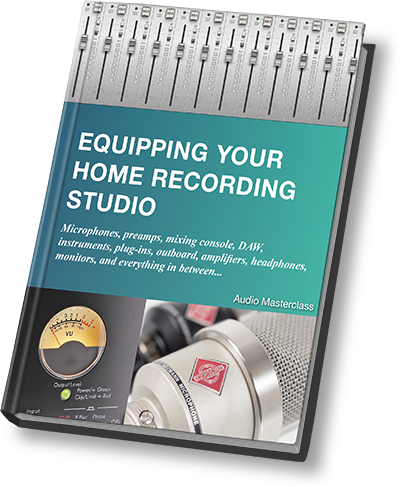# Five features of a sound wave

## All types of wave have five basic properties, and understanding of which is essential to a further grasp of acoustics...Where would we be without waves? Some physicists say we are constructed from them. In practical terms though, waves transport energy from one place to another in the form of light waves, water waves, seismic waves and of course sound waves.

Waves of all varieties have certain features in common. First is the amplitude, or 'strength', of the wave. For a water wave this would be measured in terms of the height of the wave. For sound it is measured as air pressure. For an electrical signal it is measured as voltage.

Considering a water wave since this is the easiest to visualize, the height of the wave varies in an cyclical pattern. The water goes up, then it goes down, then back up again. The distance between adjacent peaks of the wave is called the wavelength, which is measured in meters . The same goes for other types of wave. Wavelength can also be measured by the distance between two troughs, or any two similar points on the wave.

Waves always travel from one place to another, unless confined between reflecting surfaces. So if you stand at a certain point armed with a stopwatch, and measure the time interval between two successive peaks, you will have the period of the wave, which is measured in seconds. The period is the time taken between one peak to the next.

## FREE EBOOK - Equipping Your Home Recording StudioWe don't generally think in terms of period though, but its reciprocal, which is frequency, which is measured in hertz (Hz). Frequency counts how many peaks go by in one second.

The last feature of a wave is its velocity, measured in meters per second, which is simply its speed in the direction of travel. Sound travels about one foot (30 cm) in one thousandth of a second. Light travels the same distance in one millionth of a millionth of a second (1 picosecond).

Always with waves, two relationships apply:

velocity = frequency x wavelength
frequency = 1 / period

You can swap these equations round according to the rules of algebra so that...

frequency = velocity / wavelength
wavelength = velocity / frequency
period = 1 / frequency

We can use abbreviations for these quantities. Velocity is v; frequency is usually f; period is T. Wavelength is the Greek letter lamda - ?. Computers unfortunately do not work consistently with this symbol, so even though it is correctly encoded on this page, it might not display or print correctly. This applies to word processor documents too.

Understanding these basic wave properties is fundamental to a further understanding of acoustics.